# Difference between revisions of "Taylor series operator is multiplicative"

## Statement

Suppose$f$ and$g$ are functions defined on subsets of the reals such that$x_0$ is a point in the interior of the domain of both, and both$f$ and$g$ are infinitely differentiable at$x_0$. Then, the pointwise product of functions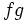$fg$ is also infinitely differentiable at$x_0$. Further, the Taylor series of$fg$ at$x_0$ is the product of the Taylor series of$f$ at$x_0$ and the Taylor series of$g$ at$x_0$.

## Facts used

1. Product rule for higher derivatives: This states that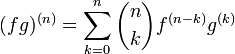$\! (fg)^{(n)} = \sum_{k=0}^n \binom{n}{k} f^{(n-k)}g^{(k)}$ wherever the right side makes sense.

## Proof

Given:$f$ and$g$ are functions defined on subsets of the reals such that$x_0$ is a point in the interior of the domain of both, and both$f$ and$g$ are infinitely differentiable at$x_0$.

To prove: The pointwise product$fg$ is infinitely differentiable at$x_0$ and its Taylor series at$x_0$ is the product of the Taylor series of$f$ at$x_0$ and the Taylor series of$g$ at$x_0$.

Proof: By Fact (1), we have:$\! (fg)^{(n)}(x_0) = \sum_{k=0}^n \binom{n}{k} f^{(n-k)}(x_0)g^{(k)}(x_0) \qquad (\dagger)$

with equality holding wherever the right side makes sense. Since the right side always makes sense, so does the left side, so$fg$ is infinitely differentiable.

We recall the expressions for the Taylor series: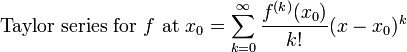$\mbox{Taylor series for } f \mbox{ at } x_0 = \sum_{k=0}^\infty \frac{f^{(k)}(x_0)}{k!}(x - x_0)^k$$\mbox{Taylor series for } g \mbox{ at } x_0 = \sum_{k=0}^\infty \frac{g^{(k)}(x_0)}{k!}(x - x_0)^k$

We now want to multiply these Taylor series. To do this, we need to determine the coefficient of$(x - x_0)^n$ in the product.$(x - x_0)^n$ can arise in the product by picking a factor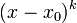$(x - x_0)^k$ from the Taylor series of$g$ and a factor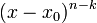$(x - x_0)^{n-k}$ from the Taylor series of$f$. The coefficient arising from such a product is$\frac{f^{(n-k)}(x_0)g^{(k)}(x_0)}{(n - k)!k!}$. The overall coefficient is thus: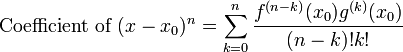$\mbox{Coefficient of } (x - x_0)^n = \sum_{k=0}^n \frac{f^{(n-k)}(x_0)g^{(k)}(x_0)}{(n - k)!k!}$

Multiply and divide the right side by$n!$ to get: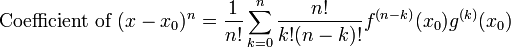$\mbox{Coefficient of } (x - x_0)^n = \frac{1}{n!} \sum_{k=0}^n \frac{n!}{k!(n- k)!} f^{(n-k)}(x_0)g^{(k)}(x_0)$

We have that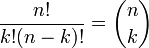$\frac{n!}{k!(n- k)!} = \binom{n}{k}$, and we get: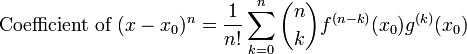$\mbox{Coefficient of } (x - x_0)^n = \frac{1}{n!} \sum_{k=0}^n \binom{n}{k} f^{(n-k)}(x_0)g^{(k)}(x_0)$

Now, using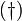$(\dagger)$, we get that: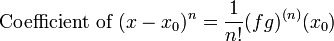$\mbox{Coefficient of } (x - x_0)^n = \frac{1}{n!} (fg)^{(n)}(x_0)$

So the product of the Taylor series for$f$ and$g$ is: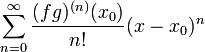$\sum_{n=0}^\infty \frac{(fg)^{(n)}(x_0)}{n!}(x - x_0)^n$

Since$n$ is a dummy variable, it can be replaced by the dummy variable$k$, giving: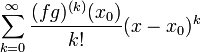$\sum_{k=0}^\infty \frac{(fg)^{(k)}(x_0)}{k!}(x - x_0)^k$

This is precisely the Taylor series for$fg$, and we have completed the proof.